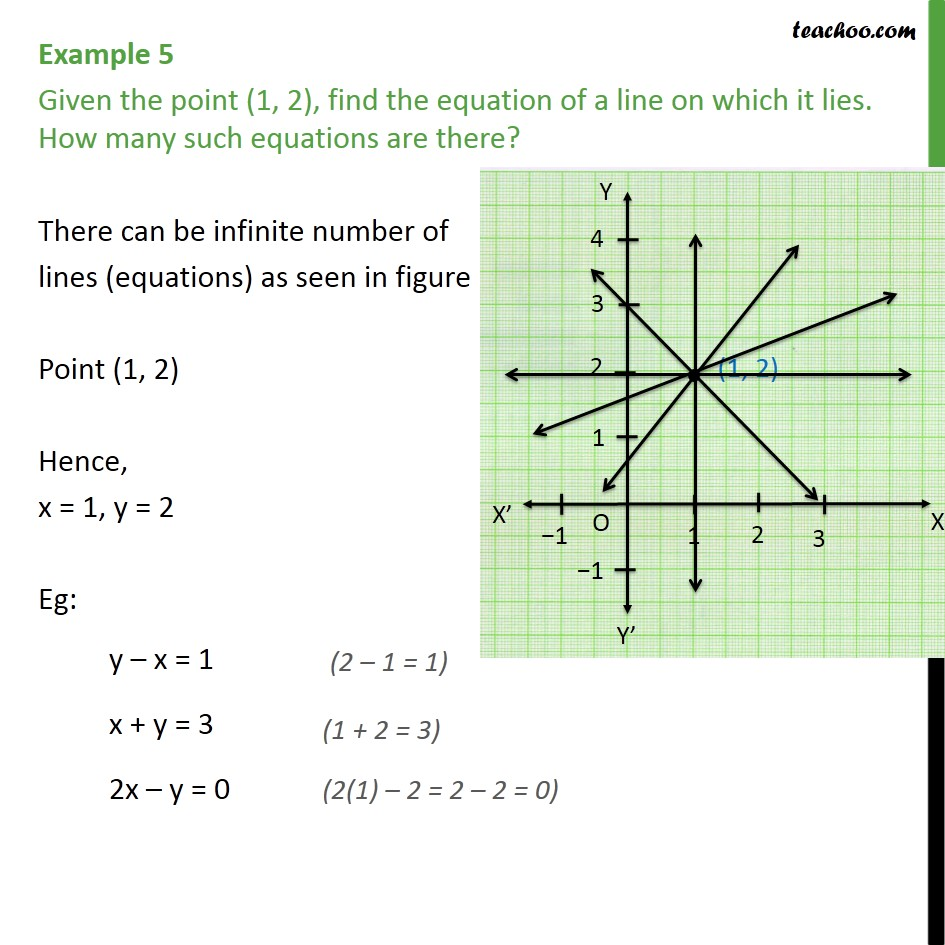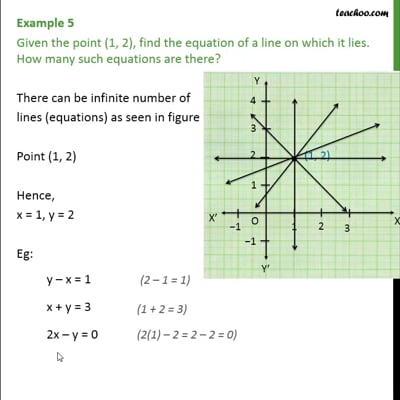Examples

Chapter 4 Class 9 Linear Equations in Two Variables
Serial order wiseThis video is only available for Teachoo black users

Solve all your doubts with Teachoo Black (new monthly pack available now!)

### Transcript

Example 5 Given the point (1, 2), find the equation of a line on which it lies. How many such equations are there? There can be infinite number of lines (equations) as seen in figure Point (1, 2) Hence, x = 1, y = 2 Eg: y – x = 1 x + y = 3 2x – y = 0